# AP Chemistry : Cell Potential Under Non-standard Conditions

## Example Questions

### Example Question #1 : Cell Potential Under Non Standard Conditions

Consider an electrochemical cell that has the following overall reaction:

2 H+(aq) + Sn (s) -> Sn2+ (aq) + H2 (aq)

Which of the following changes would alter the measured cell potential?

Lowering the pH in the cathode.

All of the above.

Increase the pH in the cathode.

Increasing the H2 pressure in the cathode.

All of the above.

Explanation: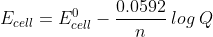All of these changes would change Q and thus change the measured cell potential.

### Example Question #2 : Cell Potential Under Non Standard Conditions

For the following cell reaction:

2 Al (s) + 3 Mn2+ (aq) -> 2 Al3+ (aq)  + 3 Mn (s)

predict if the cell potential Ecell will be larger, or smaller than Eocell for the following conditions:

[Al3+] = 2.0 M; [Mn2+] = 1.0 M.

Identical

Can not be determined

Larger

Smaller

Smaller

Explanation:Altering these conditions would increase Q, and thus result in a decrease in the measured cell potential.

### Example Question #3 : Cell Potential Under Non Standard Conditions

For the following cell reaction:

2 Al (s) + 3 Mn2+ (aq) -> 2 Al3+ (aq)  + 3 Mn (s)

predict if the cell potential Ecell will be larger, or smaller than Eocell for the following conditions:  [Al3+] = 1.0 M; [Mn2+] = 5.0 M.

Smaller

Can not be determined

Identical

Larger

Larger

Explanation:At these concentrations Q will become smaller, and thus log Q will become smaller.  This will give rise to a larger cell potential.

### Example Question #1 : Cell Potential Under Non Standard Conditions

What is the cell potential of the following cell:

Zn (s) + 2 H+(aq)  ->  Zn2+ (aq)  +  H2 (g)                                  Eo = 0.76 V

When the [Zn2+] = 1.0 M; PH2 = 1 atm, and the pH in the cathode is 5.2?

1.80 V

0.45 V

1.32 V

0.90 V

1.59 V

0.45 V

Explanation: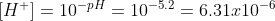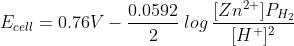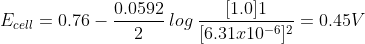### Example Question #121 : Thermochemistry And Kinetics

Calculate the standard cell potential of the following reaction:

Cd(s) +  MnO2 (s) + 4 H+ (aq) +  ->  Cd2+ (aq) +  Mn2+ (aq)  + 2 H2O (l)

Given:

MnO2 (s) + 4 H+ (aq)  + 2e-  ->  Mn2+ (aq) + 2 H2O (l)            Eo = 1.23 V

Cd2+ (aq)  + 2 e-   ->  Cd (s)                                                     Eo = -0.40 V

-0.83 V

0.83 V

-1.63 V

1.63 V

0.0 V

1.63 V

Explanation:

Eocell  = Eo cathode - Eoanode

Eocell = 1.23 – (-0.40) = 1.63 V

### Example Question #3 : Cell Potential Under Non Standard Conditions

Calculate the standard cell potential of the following reaction:

Zn (s) + Cu2+ (aq)  ->  Zn2+ (aq) + Cu (s)

Given:

Zn2+(aq)+ 2 e-->  Zn (s)                                             Eo = -0.76 V

Cu2+(aq)+ 2 e-->  Cu (s)                                             Eo = 0.34 V

0.0 V

-1.10 V

0.42 V

1.10 V

-0.42 V

1.10 V

Explanation:

Eocell  = Eo cathode - Eoanode

Eocell = 0.34 – (-0.76) = 1.10 V

### Example Question #4 : Cell Potential Under Non Standard Conditions

Calculate the standard cell potential of the following reaction:

Zn (s) + 2 Ag1+ (aq)  ->  Zn2+ (aq) + 2 Ag (s)

Given:

Zn2+(aq)+ 2 e-->  Zn (s)                                             Eo = -0.76 V

Ag1+(aq)+ 1 e-->  Ag (s)                                             Eo = 0.80 V

-1.56 V

-0.04 V

0.04 V

2.36 V

1.56 V

1.56 V

Explanation:

Eocell  = Eo cathode - Eoanode

Eocell = 0.80 – (-0.76) = 1.56 V

### Example Question #5 : Cell Potential Under Non Standard Conditions

Calculate the standard cell potential of the following reaction:

3 F2 (g) + 2 Au (s) -> 6 F- (aq) + 2 Au3+

Given:

F2 (g) + 2 e- -> 2 F- (aq)                                                  Eo = 2.87 V

Au3+(aq)+ 3 e--> Au (s)                                                  Eo = 1.50 V

5.61 V

-1.37 V

1.37 V

-5.61 V

4.37 V

1.37 V

Explanation:

Eocell  = Eo cathode - Eoanode

Eocell = 2.87 – (1.50) = 1.37 V

### Example Question #2 : Cell Potential Under Non Standard Conditions

Determine the Ecell for the following reaction at 25 C:

Zn (s) + 2 VO2+ (aq) + 4 H+ -> 2 VO2+  (aq) +  Zn2+(aq) + 2 H2O (l)

Given that:

VO2+ (aq)  +  2 H+ (aq) + e- ->  VO2+(aq) + H2O (l)        Eo = 1.00 V

Zn2+ (aq)  + 2 e-->  Zn (s)                                            Eo = -0.76 V

And

[ VO2+] = 2.0 M; [H+] = 0.50 M; [VO2+] = 1.0 x 10-2M; [Zn2+] = 1.0 x 10-1M

2.41 V

1.76 V

1.89 V

3.71 V

0.95 V

1.89 V

Explanation: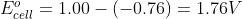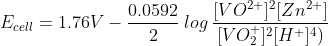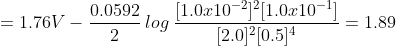### All AP Chemistry Resources